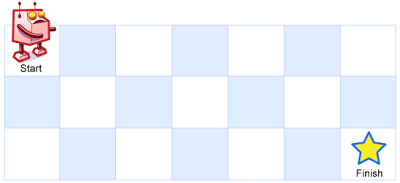2019/04/10 10:10

# 动态规划

## 动态规划四步走

• **明确数组的含义：**建立数组，明确数组元素的含义；
• **制作历史记录表：**根据数组建表，填入初始值，利用递推关系式写程序推出其他空表项。

**注意：**这个是为我们通过初始值和递推关系式写出程序提供可视化条件以及思路，把抽象的东西可视化了，时时刻刻都知道自己要干嘛。 当然，如果脑子里有思路可以忽略。。

**启发：**这个想法其实是由编译原理中的 “编译程序绝大多数时间花在管理表格上” 这句话来的。 因为在编译中，编译的每个阶段所需要的信息多数从表格中读取，产生的中间结果都记录在相应的表格中，可以说整个编译过程就是造表、查表的过程。 也就是说，我们的动态规划算法也可以理解成造表、查表的一个过程。

• **寻找数组初始值：**寻找数组元素初始值；

• **找出递推关系式：**找出数组元素递推关系式。

**注意：**可以从 dp[i] = ? 这一数学公式开始推想。

### 明确数组的含义

**第一步：**定义数组元素的含义。 上面说了，我们会用一个数组，来保存历史记录，假设用一维数组 dp[]吧。这个时候有一个非常非常重要的点，就是规定你这个数组元素的含义，即 你的 dp[i] 是代表什么意思？

### 制作历史记录表

1. 根据数组，制表，确定一维表、二维表；
2. 填入初始值
3. 根据递推关系式，写程序推出剩余的空表项

**注意：**这里一维表比较简单可能体现不出它的作用，到二维表它就能很方便的将数据可视化了。

### 寻找数组初始值

**第二步：**找出初始值。 利用我们学过数学归纳法，我们可以知道如果要进行递推，我们需要一个初始值来推出结果值，也就是我们常说的第一张多米诺骨牌。

• 当 n = 1 时，即 只有一级台阶，那么我们的青蛙只用跳一级就可以了，只有一种跳法，dp = 1；
• 当 n = 2 时，即 有两级台阶，我们的青蛙有两种选择，一级一级的跳 和 一次跳两级，dp = 2；
• 当 n = 3 时，即 有三级台阶，我们的青蛙跳一级 + dp，或 跳两级 + dp，这时候我们就反应过来了，需要进行下一步找出 n 的递推关系式。

1 2

### 找出递推关系式

n = i 时，即 有 i 级台阶，我们的青蛙最后究竟怎么样到达的这第 i 级台阶呢？ 因为青蛙的弹跳力有限，只能一次跳一级或者两级，所以我们有两种方式可以到达最后的这第 i 级：

• 从 i-1 处跳一级
• 从 i-2 处跳两级

1 2 3

// 青蛙跳台阶
int f(int n) {
// 特别给初始值的出口
if(n <= 2)
return n;

// 创建数组保存历史数据
int[] dp = new int[n+1];

// 给出初始值
dp = 1;
dp = 2;

// 通过递推关系式来计算出 dp[n]
for(int i = 3; i <= n; i++) {
dp[i] = dp[i-1] + dp[i-2];
}

// 把最终结果返回
return dp[n];
}


# 实例

## 超级青蛙跳台阶

Fib(n) 表示超级青蛙🐸跳上 n 阶台阶的跳法数。 如果按照定义，Fib(0)肯定需要为 0，否则没有意义。我们设定 Fib(0) = 1；n = 0 是特殊情况，通过下面的分析会知道，令 Fib(0) = 1 很有好处。

**PS：**Fib(0)等于几都不影响我们解题，但是会影响我们下面的分析理解。

• 当 n = 1 时， 只有一种跳法，即 1 阶跳：$Fib(1) = 1$;

• 当 n = 2 时， 有两种跳的方式，一阶跳和二阶跳：$Fib(2) = 2$; 到这里为止，和普通跳台阶是一样的。

• 当 n = 3 时，有三种跳的方式，第一次跳出一阶后，对应 Fib(3-1) 种跳法； 第一次跳出二阶后，对应 Fib(3-2)种跳法；第一次跳出三阶后，只有这一种跳法。 $Fib(3) = Fib(2) + Fib(1)+ 1 = Fib(2) + Fib(1) + Fib(0) = 4$

• 当 n = 4 时，有四种方式：第一次跳出一阶，对应 Fib(4-1)种跳法；第一次跳出二阶，对应Fib(4-2)种跳法；第一次跳出三阶，对应 Fib(4-3)种跳法；第一次跳出四阶，只有一种跳法。 $Fib(4) = Fib(4-1) + Fib(4-2) + Fib(4-3) + 1 = Fib(4-1) + Fib(4-2) + Fib(4-3) + Fib(4-4)$

• 当 n = n 时，共有 n 种跳的方式： 第一次跳出一阶后，后面还有 Fib(n-1)中跳法； ... ... ... 第一次跳出 n 阶后，后面还有 Fib(n-n)中跳法。 $Fib(n) = Fib(n-1)+Fib(n-2)+Fib(n-3)+...+Fib(n-n) = Fib(0)+Fib(1)+Fib(2)+...+Fib(n-1)$

public class SY1 {
//自底向上的动态规划 超级青蛙 N 阶跳
static long solution(int number) {
//题目保证 number 最大为 50
long[] Counter=new long;
Counter = 1;
Counter = 1;
Counter = 2;
int calculatedIndex = 2;
if(number <= calculatedIndex)
return Counter[number];
if(number > 50) //防止下标越界
number = 50;
for(int i = calculatedIndex + 1; i <= number; i++)
Counter[i] = 2 * Counter[i - 1];
calculatedIndex = number;
return Counter[number];
}
public static void main(String[] args) {
Scanner cin = new Scanner(System.in);
System.out.print(solution(cin.nextInt()));
}
}


## 不同路径

• 机器人每次只能向下或者向右移动一步。例如，上图是一个 3 x 7 的网格。有多少可能的路径？

0 1 2 m
0
1
2
n

### 寻找数组初始值

• dp[0…n-1] = 1; // 第一行，机器人只能一直往右走
• dp[0…m-1] = 1; // 第一列，机器人只能一直往下走

0 1 2 m
0 1 1 1 1
1 1
2 1
n 1

### 找出递推关系式

• 一种是从 (i-1, j) 这个位置走一步到达
• 一种是从 (i, j-1) 这个位置走一步到达

0 1 2 m
0 1 1 1 1
1 1 2 3
2 1 3 6
n 1

public static int uniquePaths(int m, int n) {
if (m <= 0 || n <= 0) {
return 0;
}

int[][] dp = new int[m][n];	// 地图

// 初始化
for(int i = 0; i < m; i++) {
dp[i] = 1;
}
for(int i = 0; i < n; i++) {
dp[i] = 1;
}

// 递推 dp[m-1][n-1]
for (int i = 1; i < m; i++) {	// 逐行填写空表格
for (int j = 1; j < n; j++) {
dp[i][j] = dp[i-1][j] + dp[i][j-1];
}
}
return dp[m-1][n-1];
}### 作者的其它热门文章

0
0 收藏

0 评论
0 收藏
0